﻿ Numerical Solution of Fredholm Integral Equations Using Hosoya Polynomial of Path GraphsPublications are Open
Access in this journal
Article Versions
Export Article
• Normal Style
• MLA Style
• APA Style
• Chicago Style
Research Article
Open Access Peer-reviewed

### Numerical Solution of Fredholm Integral Equations Using Hosoya Polynomial of Path Graphs

H. S. Ramane, S.C. Shiralashetti, R. A. Mundewadi , R. B. Jummannaver
American Journal of Numerical Analysis. 2017, 5(1), 11-15. DOI: 10.12691/ajna-5-1-2
Published online: January 09, 2018

### Abstract

The main purpose of this paper is to develop the graph theoretic polynomial to solve numerical problems. We present a new method for the solution of Fredholm integral equations using Hosoya polynomials obtained from one of the standard class of graphs called as path. Proposed algorithm expands the desired solution in terms of a set of continuous polynomials over a closed interval [0,1]. However, accuracy and efficiency are dependent on the size of the set of Hosoya polynomials and compared with the existing method.

### 1. Introduction

In graph theory, as in discrete mathematics in general, not only the existence, but also the counting of objects with some given properties, is of main interest. Each area introduces its own special terms for shared concepts in discrete mathematics. The only way to keep from reinventing the wheel from area to area is to know the precise mathematical ideas behind the concept being applied by these various fields. Graph theory is rapidly moving into the mainstream of mathematics mainly because of its applications in diverse fields which include biochemistry, electrical engineering (Communications networks and coding theory), computer science (algorithms and computations) and operations research (scheduling) 1, 2.

Integral equations have motivated a large amount of research work in recent years. Integral equations find its applications in various fields of mathematics, science and technology has been studied extensively both at the theoretical and practical level. In particular, integral equations arise in fluid mechanics, biological models, solid state physics, kinetics in chemistry etc. In most of the cases, it is difficult to solve them, especially analytically 3. Analytical solutions of integral equations, however, either does not exist or are difficult to find. It is precisely due to this fact that several numerical methods have been developed for finding solutions of integral equations.

Consider the Fredholm integral equation: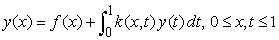(1)

where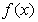and the kernels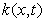are assumed to be in L2(R) on the interval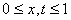. We assume that Eq.(1) has a unique solution y to be determined. There are several numerical methods for approximating the solution of Fredholm integral equations are known and many different basic functions have been used. Such as, Lepik et al. 4 applied the Haar Wavelets. Maleknejad et al. 5 applied a combination of Hybrid taylor and block-pulse functions, Rationalized Haar wavelet 6, Hermite Cubic splines 7. Muthuvalu et al. 8 applied Half-sweep arithmetic mean method with composite trapezoidal scheme for the solution of Fredholm integral equations. In this paper, we proposed a numerical method for the solution of Fredholm integral equations using Hosoya polynomial of paths as basis.

### 2. Properties of Hosoya Polynomial

A graph G consists of a finite nonempty set V of n points (vertices) together with a prescribed set X of m unordered pairs of distinct points of V. Each pair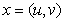of points in X is an edge of G. If the points u and v are joined by an edge, then we say that u and v are adjacent points. Let v1, v2, … ,vn be the vertices of G. The path Pn is a graph with n vertices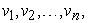where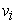is adjacent to vi + 1, i = 1, 2, …, n – 1. The length of a path is the number of edges in it. A graph G is said to be connected if every pair of points of G is joined by some path. The distance between the verticesand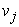in G is equal to the length of the shortest path joining them and is denoted by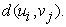For more details about the graph theory one can refer the book 9.

The Wiener index W(G) of a connected graph G is defined as the sum of the distances between all unordered pairs of vertices of G, that is,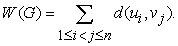This index was put forward by Harold Wiener 10 in 1947 for approximation of the boiling points of alkanes. The effect of approximation was surprisingly good. From that point forward, the Wiener index has attracted the attention of chemists.

The Hosoya polynomial of a graph is a generating function about distance distributing, introduced by Hosoya 11 in 1988. For a connected graph G, the Hosoya polynomial denoted by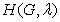is defined as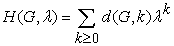(2)

where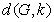is the number of pairs of vertices of G that are at distance k and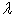is the parameter.

The connection between the Hosoya polynomial and the Wiener index is elementary 11, 12: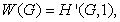where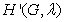is the first derivative of.

Hosoya polynomial of tress 13, 14, composite graphs 15, benzenoid graphs 16, 17, tori 18, zig-zag open-ended nanotubes 19, armchair open-ended nanotubes 20, zigzag polyhexnanotorus 21, Fibonacci and Lucas cubes 22 are reported in the literature.

The paths P1, P2 and P3 are depicted in Figure 1.

• Figure 1. Path graphs P1, P2 and P3

The Hosoya polynomial of a path Pn is: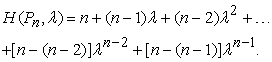In particular,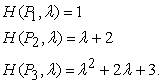### 3. Function Approximation

A function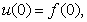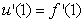is expanded as: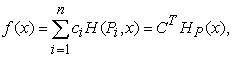(3)

where C and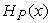are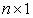matrices given by: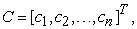(4)

and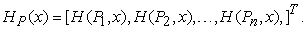(5)

### 4. Hosoya Polynomial Method (HPM)

Consider the Fredholm integral equation,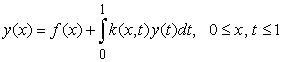(6)

To solve Eq. (6), the procedure is as follows:

Step 1: We first approximate y(x) as truncated series defined in Eq. (3). That is,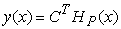(7)

where C andare defined as in Eqs. (4) and (5).

Step 2: Substituting Eq. (7) in Eq. (6), we get,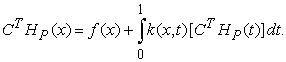(8)

Step 3: Substituting the collocation point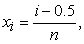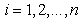in Eq. (8), we obtain,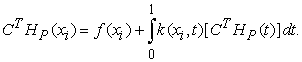(9)

That is,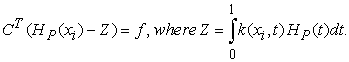Step 4: Now, we get the system of algebraic equations with unknown coefficients.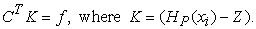Solving the above system of equations, we get the Hosoya coefficients ‘C’ and then substituting these coefficients in Eq. (7), we get the required approximate solution of Eq. (6).

### 5. Numerical Results

In this section, we consider the some illustrative examples from the literature to demonstrate the capability of the method and error function is presented to verify the accuracy and efficiency of the numerical results: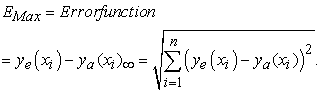where,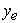and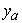are the exact and approximate solution respectively.

We considered the examples given in 7. Using the present technique, the numerical solutions with exact solutions presented in Table 1 and the maximum error analysis compared with existing method 7 given in Table 2.

Example 1. Consider Fredholm integral equations,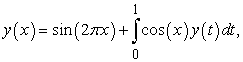(10)

which has the exact solution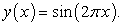Solution: First we substitutein Eq. (10) we get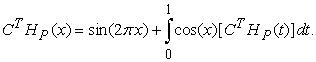Therefore for n = 3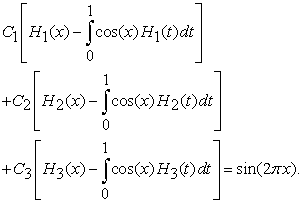Next, we substitute the Hosoya polynomials as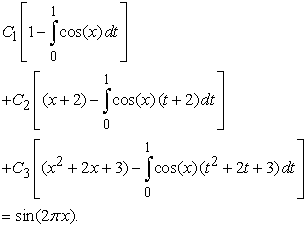Next,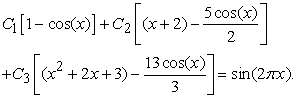Substituting the collocation points, we get the system of three equations with three unknowns as,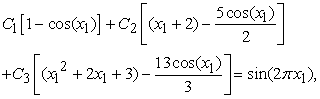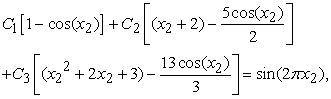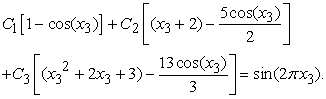Solving these systems we obtain the three unknown Hosoya coefficients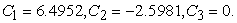Substituting these coefficients in the approximation,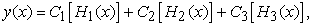we get the approximate values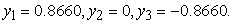Maximum Error analyzed for n = 3 is 1.55e-15 and for n = 4, 6 & 8 are shown in the Table 2.

Example 2. Consider Fredholm integral equations,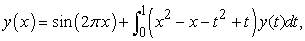(11)

has the exact solution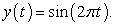Example 3. Consider Fredholm integral equations,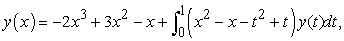(12)

which has the exact solution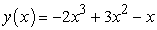.

• Figure 2. Numerical solution of exact and HPM Example 1, for n = 8
• Figure 3. Numerical solution of exact and HPM Example 2, for n = 8
• Figure 4. Numerical solution of exact and HPM Example 3, for n = 8

### 6. Conclusion

The Hosoya polynomial method is applied for the numerical solution of Fredholm integral equations. The present method reduces an integral equation into a set of algebraic equations. For instance in Example 1, our results are higher accuracy with exact ones and existing method 7. Subsequently other examples are also same in the nature. The numerical result shows that the accuracy improves with increasing of n, the order of a path Pn , for better accuracy. Error analysis justifies the accuracy, efficiency and validity of the present technique.

### References

  L. Caccetta, K. Vijayan, Applications of graph theory, Ars. Combin., 23 (1987), 21-77. In article View Article  F. S. Roberts, Graph Theory and Its Applications to the Problems of Society, SIAM Publications, Philadelphia, 1978. In article View Article  A.M. Wazwaz. A First Course in Integral Equations. WSPC. New Jersey. 1997. In article View Article  U. Lepik, E. Tamme, Application of the Haar wavelets for solution of linearintegral equations, in: Dynamical Systems and Applications, Antala. Proce. (2004). 494-507. In article View Article  K. Maleknejad, Y. Mahmoudi, Numerical solution of linear Fredholm integral equation by using hybrid Taylor and Block-Pulse functions, App.Math. Comp. 149 (2004). 799-806. In article View Article  K. Maleknejad, F. Mirzaee, Using rationalized Haar wavelet for solvinglinear integral equations, App. Math. Comp. 160 (2005). 579-587. In article View Article  K. Maleknejad, M. Yousefi, Numerical solution of the integral equation ofthe second kind by using wavelet bases of hermite cubic splines, App. Math.Comp. 183 (2006). 134-141. In article View Article  M. S. Muthuvalu, J. Sulaiman, Half-Sweep Arithmetic Mean method withcomposite trapezoidal scheme for solving linear fredholm integral equations, App. Math. Comp. 217 (2011). 5442-5448. In article View Article  F. Harary, Graph Theory, Addison Wesley, Reading, 1968. In article  H. Wiener, Structural determination of paraffin boiling points, J. Amer. Chem. Soc., 69 (1947), 17-20. In article View Article  H. Hosoya, On some counting polynomials in chemistry, Discrete Appl.Math., 19 (1988), 239-257. In article View Article  E. V. Konstantinova, M. V. Diudea, The Wiener polynomial derivativesand other topological indices in chemical research, Croat. Chem. Acta, 73 (2000), 383-403. In article View Article  D. Stevanovic, I. Gutman, I. Hosoya polynomials of trees with up to 11vertices, Zb. Rad. (Kragujevac), 21 (1999), 111-119. In article  H. B. Walikar, H. S. Ramane, L. Sindagi, S. S. Shirkol, I. Gutman, Hosoya polynomial of thorn trees, rods, rings and stars, Kragujevac J. Sci., 28(2006), 47-56. In article View Article  D. Stevanovic, Hosoya polynomial of composite graphs, Discrete Math., 235 (2001), 237-244. In article View Article  I. Gutman, S. Klavzar, M. Petkovšek, P. Žigert, On Hosoya polynomials of benzenoid graphs, MATCH Commun. Math. Comput. Chem., 43 (2001), 49-66. In article View Article  S. Xu, H. Zhang, The Hosoya polynomial decomposition for cata condensed benzenoid graphs, Discrete Appl. Math., 156 (2008), 2930-2938. In article View Article  M. V. Diudea, Hosoya polynomial in tori, MATCH Commun. Math. Comput. Chem., 45 (2002), 109-122. In article  S. Xu, H. Zhang, M. V. Diudea, Hosoya polynomials of zig-zag open-ended nanotubes, MATCH Commun. Math. Comput. Chem., 57 (2007), 443-456. In article View Article  S. Xu, H. Zhang, Hosoya polynomials of armchair open-ended nanotubes, Int. J. Quantum Chem., 107 (2007), 586-596. In article View Article  M. Eliasi, B. Taeri, Hosoya polynomial of zigzag polyhexnanotorus, J.Serb. Chem. Soc., 73 (2008), 311-319. In article View Article  S. Klavzar, M. Mollard, Wiener index and Hosoya polynomial of Fibonacci and Lucas cubes, MATCH Commun. Math. Comput. Chem., 68 (2012), 311-324. In article View Article

Published with license by Science and Education Publishing, Copyright © 2017 H. S. Ramane, S.C. Shiralashetti, R. A. Mundewadi and R. B. JummannaverThis work is licensed under a Creative Commons Attribution 4.0 International License. To view a copy of this license, visit http://creativecommons.org/licenses/by/4.0/